## Lamport

On October 18th 1979, Leslie Lamport published his concept of One Time Signatures.

Most signature schemes rely in part on one-way functions, typically hash functions, for their security proofs. The beauty of Lamport scheme was that this signature was only relying on the security of these one-way functions.here you have a very simple scheme, where $$x$$ and $$y$$ are both integers, and to sign a single bit:

• if it’s $$0$$, publish $$x$$

• if it’s $$1$$, publish $$y$$

Pretty simple right? Don’t use it to sign twice obviously.

Now what happens if you want to sign multiple bits? What you could do is hash the message you want to sign (so that it has a predictible output length), for example with SHA-256.

Now you need 256 private key pairs: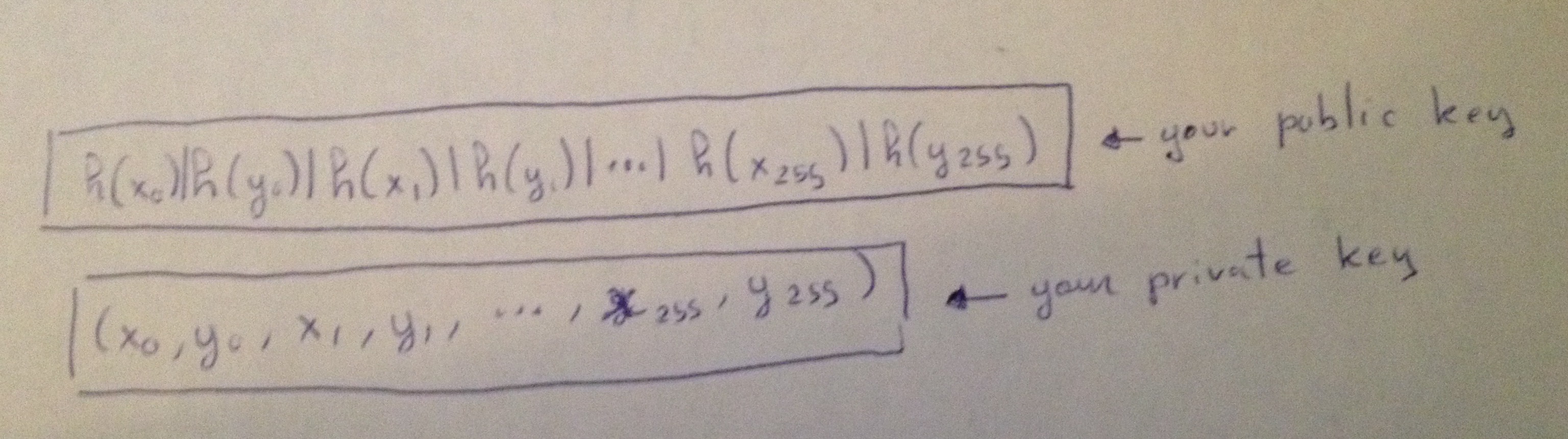and if you want to sign $$100110_2 \dots$$,

you would publish $$(y_0,x_1,x_2,y_3,y_4,x_5,\dots)$$

## Winternitz OTS (WOTS)

A few months after Lamport’s publication, Robert Winternitz of the Stanford Mathematics Department proposed to publish $$h^w(x)$$ instead of publishing $$h(x)|h(y)$$.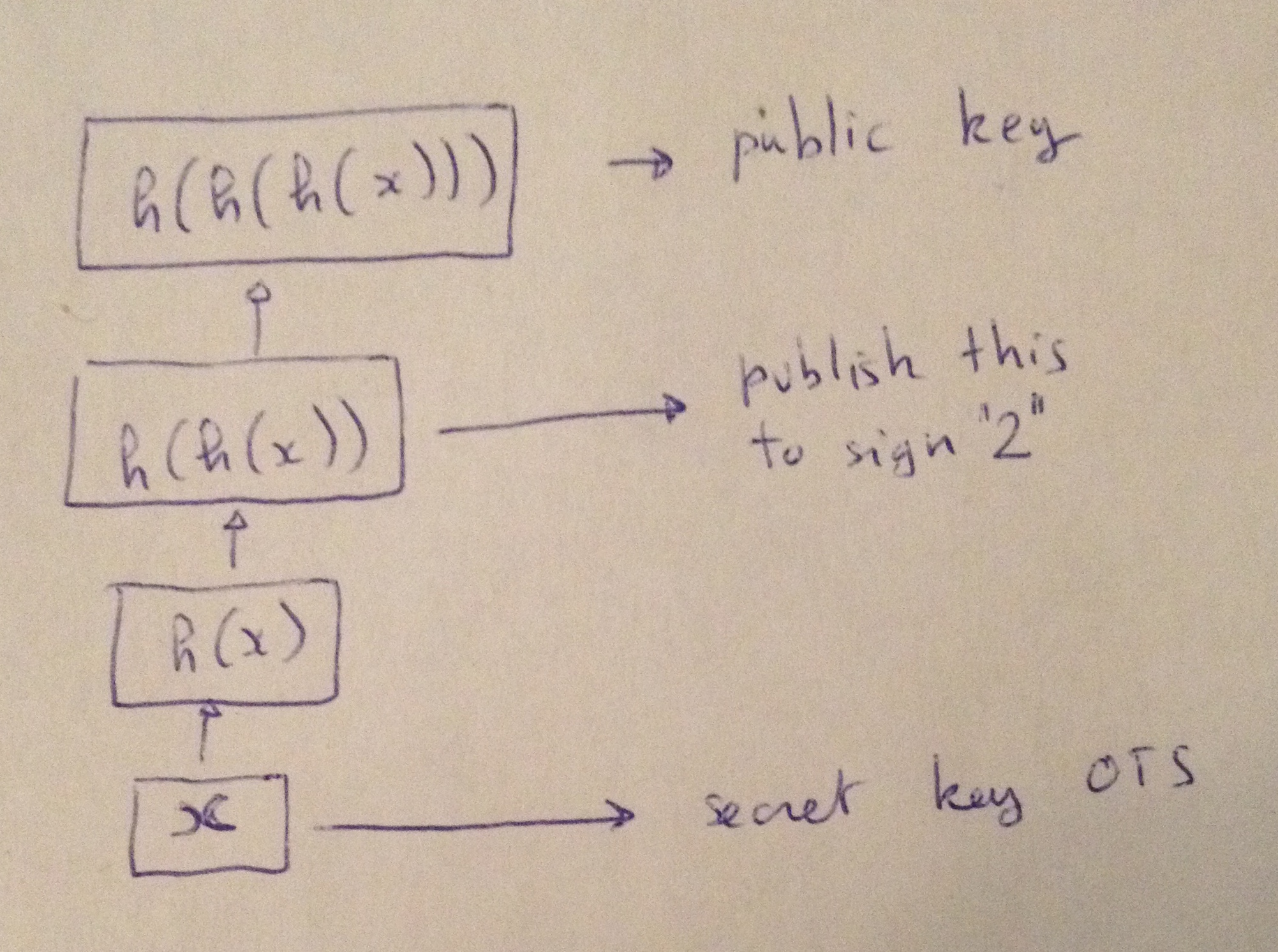For example you could choose $$w=16$$ and publish $$h^{16}(x)$$ as your public key, and $$x$$ would still be your secret key. Now imagine you want to sign the binary $$10012$$ ($$9{10}$$), just publish $$h^9(x)$$.

Another problem now is that a malicious person could see this signature and hash it to retrieve $$h^{10}(x)$$ for example and thus forge a valid signature for $$10102$$ ($$10{10}$$).

This can be circumvented by adding a short Checksum after the message (which you would have to sign as well).

## Variant of Winternitz OTS

A long long time after, in 2011, Buchmann et al published an update on Winternitz OTS and introduced a new variant using families of functions parameterized by a key. Think of a MAC.

Now your private key is a list of keys that will be use in the MAC, and the message will dictates how many times we iterate the MAC. It’s a particular iteration because the previous output is replacing the key, and we always use the same public input. Let’s see an example: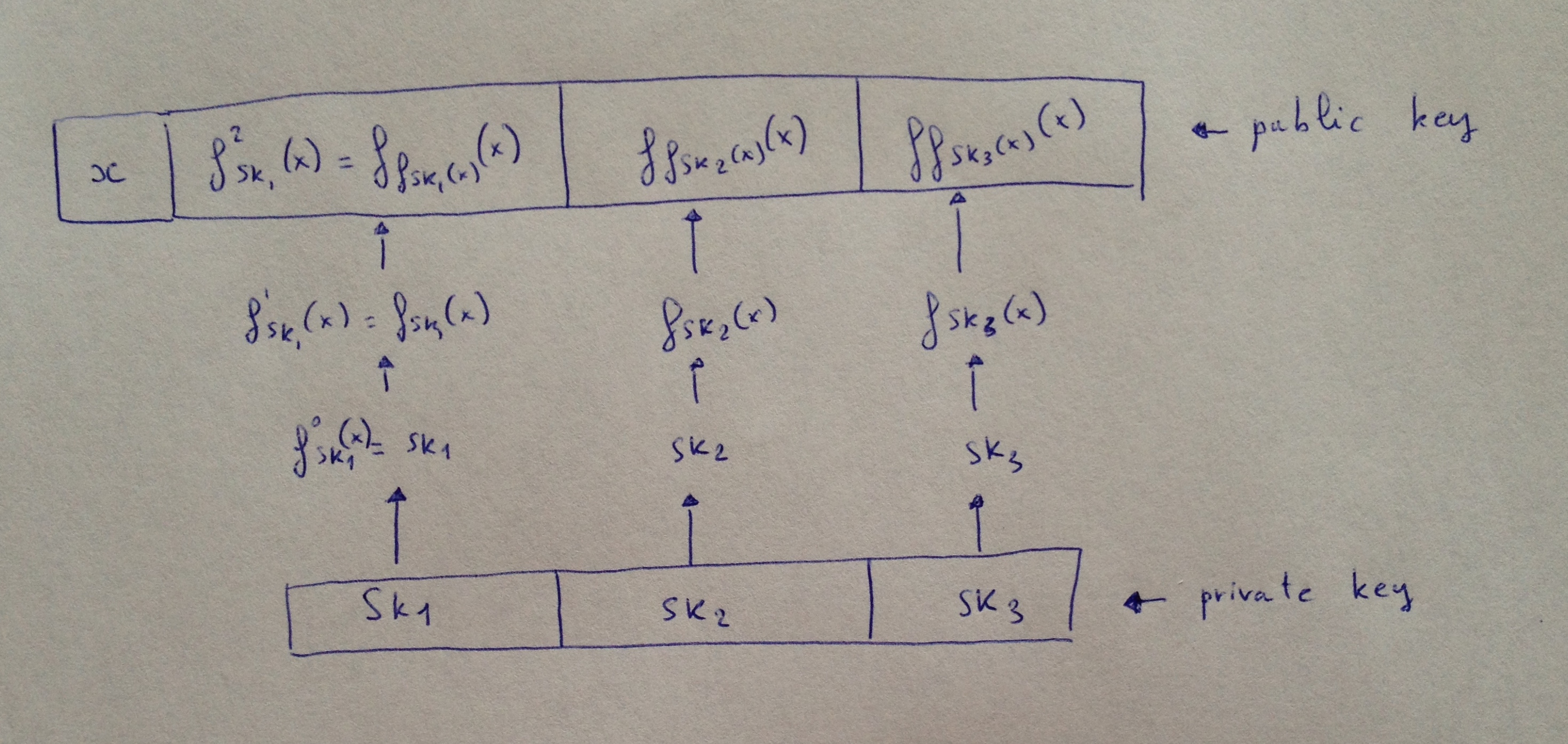We have a message $$M = 10112 (= 11{10})$$ and let’s say our variant of W-OTS works for messages in base 3 (in reality it can work for any base $$w$$). So we’ll say $$M = (M_0, M_1, M_2) = (1, 0, 2)$$ represents $$102_3$$.

To sign this we will publish $$(f_{sk_1}(x), sk_2, f^2{sk_3}(x) = f{f_{sk_3}(x)}(x))$$

Note that I don’t talk about it here, but there is still a checksum applied to our message and that has to be signed. This is why it doesn’t matter if the signature of $$M_2 = 2$$ is already known in the public key.

Intuition tells me that a public key with another iteration would provide better security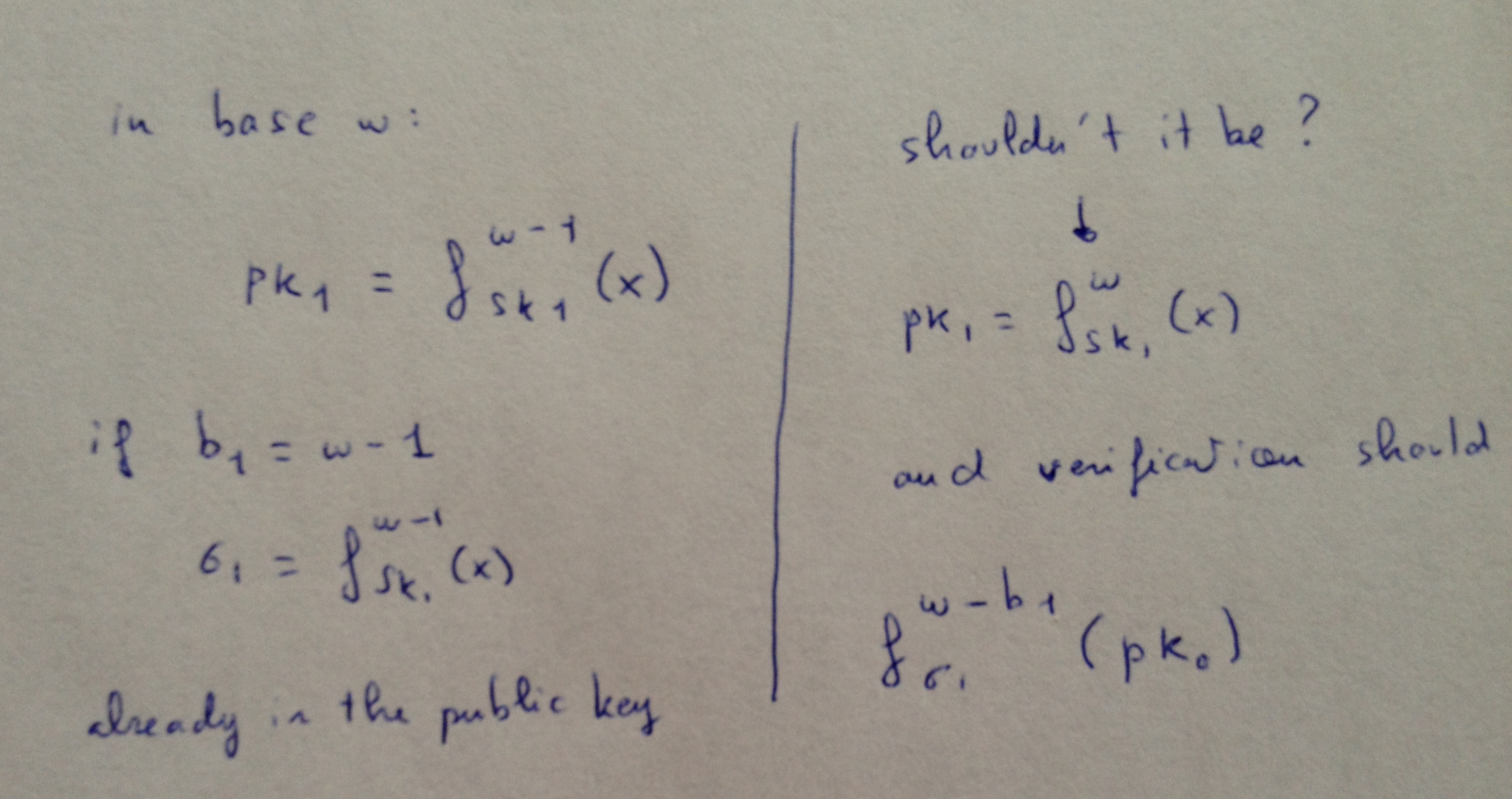here’s Andreas Hulsing’s answer after pointing me to his talk on the subject:

Why? For the 1 bit example: The checksum would be 0. Hence, to sign that message one needs to know a preimage of a public key element. That has to be exponentially hard in the security parameter for the scheme to be secure. Requiring an attacker to be able to invert the hash function on two values or twice on the same value only adds a factor 2 to the attack complexity. That’s not making the scheme significantly more secure. In terms of bit security you might gain 1 bit (At the cost of ~doubling the runtime).

## Winternitz OTS+ (WOTS+)

There’s not much to say about the W-OTS+ scheme. Two years after the variant, Hulsing alone published an upgrade that shorten the signatures size and increase the security of the previous scheme. It uses a chaining function in addition to the family of keyed functions. This time the key is always the same and it’s the input that is fed the previous output. Also a random value (or mask) is XORed before the one-way function is applied.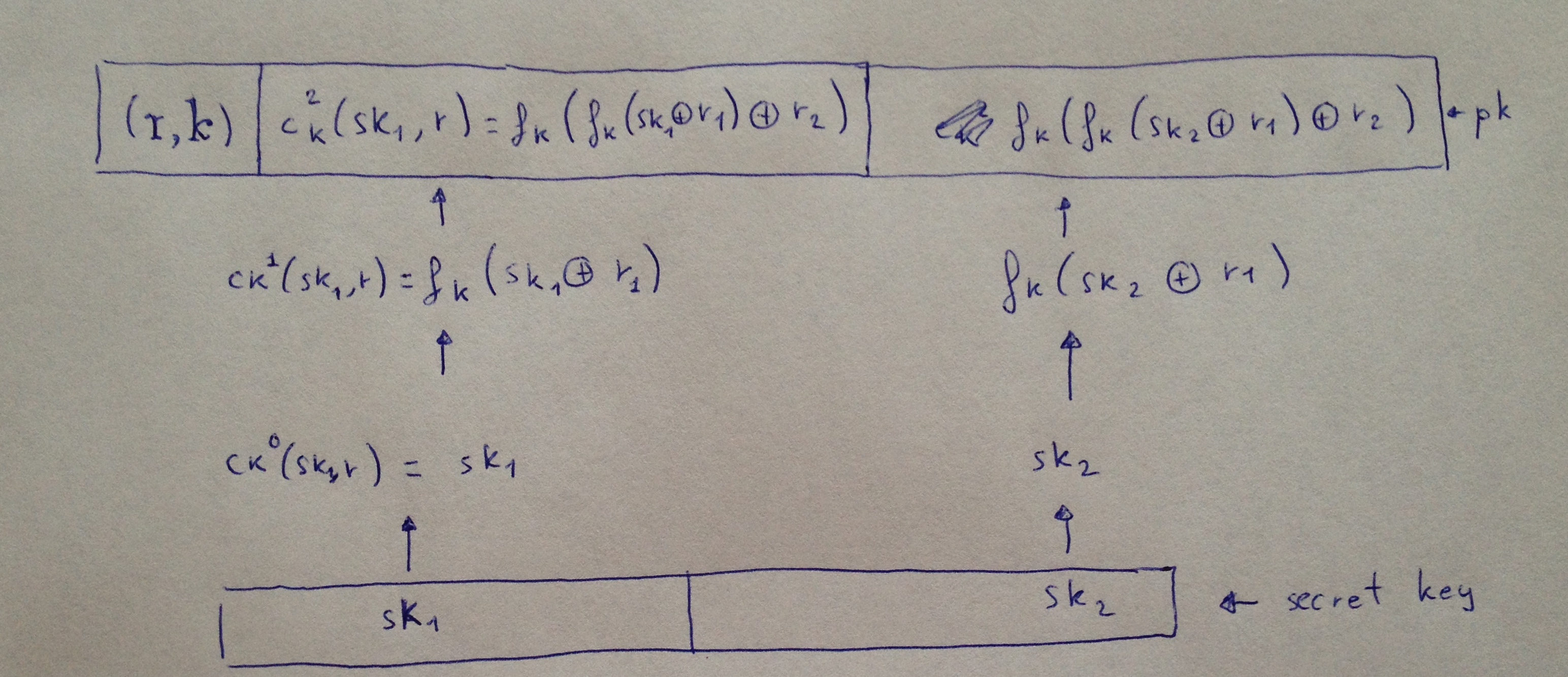Some precisions from Hulsing about shortening the signatures size:

WOTS+ reduces the signature size because you can use a hash function with shorter outputs than in the other WOTS variants at the same level of security or longer hash chains. Put differently, using the same hash function with the same output length and the same Winternitz parameter w for all variants of WOTS, WOTS+ achieves higher security than the other schemes. This is important for example if you want to use a 128 bit hash function (remember that the original WOTS requires the hash function to be collision resistant, but our 2011 proposal as well as WOTS+ only require a PRF / a second-preimage resistant hash function, respectively). In this case the original WOTS only achieves 64 bits of security which is considered insecure. Our 2011 proposal and WOTS+ achieve 128 - f(m,w) bits of security. Now the difference between WOTS-2011 and WOTS+ is that f(m,w) for WOTS-2011 is linear in w and for WOTS+ it is logarithmic in w.

## Other OTS

Here ends today’s blogpost! There are many more one-time signature schemes, if you are interested here’s a list, some of them are even more than one-time signatures because they can be used a few times. So we can call them few-times signatures schemes (FTS):

So far their applications seems to be reduce to be the basis of Hash-based signatures that are the current advised signature scheme for post quantum usage. See PQCrypto initial recommendations that was released a few months ago.

PS: Thanks to Andreas Hulsing for his comments

Part II of this series is here